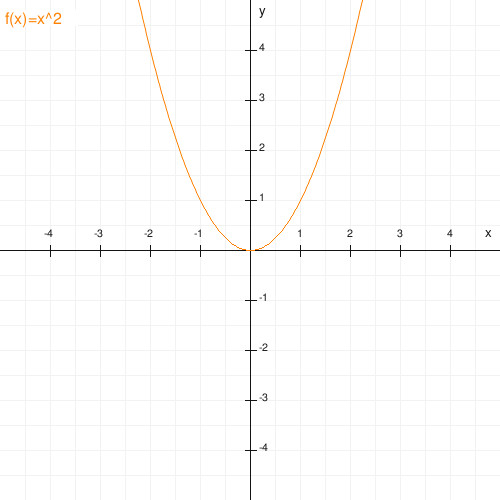### Plotter

is an online function plotter based on PHP (and a tiny bit of JavaScript). Use it to draw curves of many mathematical functions (or expressions) as an image in jpeg, png or gif format. It is simple to use and produces nice graphics by default but can do some quite complex graphs with a bit of an effort. At the moment it is limited to cartesian coordinates (a logarithmic scale is possible).These are a jpeg and a png image created dynamically by my plotter. The tool knows many interesting functions used in mathematics, statistics and even physics, can draw images of their curves on demand and calculate tables of function values (there's a latex tabular format).

### Why and how

plotter was created because I'm lazy - I wanted the possibility to do quick and (more or less) painless plots of functions to use in some advanced maths lectures.

To run a plotter, a webserver with PHP >= 4.3 and an enabled GD-Extension is required.